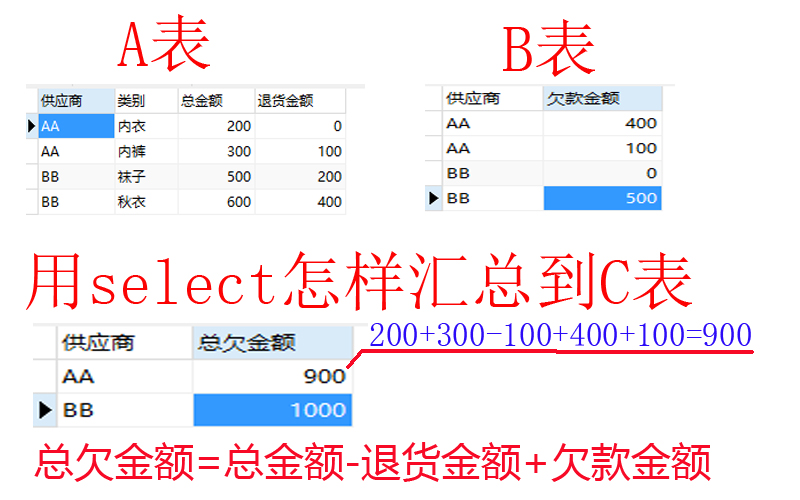4个回答

select count（a.总金额）-count（a.退货金额）+（b.欠款金额） from A表 as a inner join B表 as b where a.供应商 =b.供应商 group by a.供应商 应该可以得到你想要的结果 试下吧

select （sumTable.总金额+sumTable.欠款金额-sumTable.退回金额） from (
select sum(a.总金额) as 总金额,sum(a.退货金额) as 退货金额,sum(b.欠款金额) as欠款金额 from a left join b on a.供应商=b.供应商 group by 供应商
) sumTable
,不知道能不能在列上面加减，没试过，如果可以的话上面逻辑应该可以